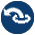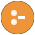#aggregate.pl -- Aggregation operators on backtrackable predicates

This library provides aggregating operators over the solutions of a predicate. The operations are a generalisation of the bagof/3, setof/3 and findall/3 built-in predicates. Aggregations that can be computed incrementally avoid findall/3 and run in constant memory. The defined aggregation operations are counting, computing the sum, minimum, maximum, a bag of solutions and a set of solutions. We first give a simple example, computing the country with the smallest area:

```smallest_country(Name, Area) :-
aggregate(min(A, N), country(N, A), min(Area, Name)).```

There are four aggregation predicates (aggregate/3, aggregate/4, aggregate_all/3 and aggregate/4), distinguished on two properties.

aggregate vs. aggregate_all
The aggregate predicates use setof/3 (aggregate/4) or bagof/3 (aggregate/3), dealing with existential qualified variables (`Var^Goal`) and providing multiple solutions for the remaining free variables in Goal. The aggregate_all/3 predicate uses findall/3, implicitly qualifying all free variables and providing exactly one solution, while aggregate_all/4 uses sort/2 over solutions that Discriminator (see below) generated using findall/3.
The Discriminator argument
The versions with 4 arguments deduplicate redundant solutions of Goal. Solutions for which both the template variables and Discriminator are identical will be treated as one solution. For example, if we wish to compute the total population of all countries, and for some reason `country(belgium, 11000000)` may succeed twice, we can use the following to avoid counting the population of Belgium twice:
`    aggregate(sum(P), Name, country(Name, P), Total)`

All aggregation predicates support the following operators below in Template. In addition, they allow for an arbitrary named compound term, where each of the arguments is a term from the list below. For example, the term `r(min(X), max(X))` computes both the minimum and maximum binding for X.

count
Count number of solutions. Same as `sum(1)`.
sum(Expr)
Sum of Expr for all solutions.
min(Expr)
Minimum of Expr for all solutions.
min(Expr, Witness)
A term `min(Min, Witness)`, where Min is the minimal version of Expr over all solutions, and Witness is any other template applied to solutions that produced Min. If multiple solutions provide the same minimum, Witness corresponds to the first solution.
max(Expr)
Maximum of Expr for all solutions.
max(Expr, Witness)
As `min(Expr, Witness)`, but producing the maximum result.
set(X)
An ordered set with all solutions for X.
bag(X)
A list of all solutions for X.

Acknowledgements

The development of this library was sponsored by SecuritEase, http://www.securitease.com

Compatibility
- Quintus, SICStus 4. The forall/2 is a SWI-Prolog built-in and term_variables/3 is a SWI-Prolog built-in with different semantics.
To be done
- Analysing the aggregation template and compiling a predicate for the list aggregation can be done at compile time.
- aggregate_all/3 can be rewritten to run in constant space using non-backtrackable assignment on a term.
aggregate(+Template, :Goal, -Result) is nondet
Aggregate bindings in Goal according to Template. The aggregate/3 version performs bagof/3 on Goal.
aggregate(+Template, +Discriminator, :Goal, -Result) is nondet
Aggregate bindings in Goal according to Template. The aggregate/4 version performs setof/3 on Goal.
aggregate_all(+Template, :Goal, -Result) is semidet
Aggregate bindings in Goal according to Template. The aggregate_all/3 version performs findall/3 on Goal. Note that this predicate fails if Template contains one or more of `min(X)`, `max(X)`, `min(X,Witness)` or `max(X,Witness)` and Goal has no solutions, i.e., the minimum and maximum of an empty set is undefined.

The Template values `count`, `sum(X)`, `max(X)`, `min(X)`, `max(X,W)` and `min(X,W)` are processed incrementally rather than using findall/3 and run in constant memory.

aggregate_all(+Template, +Discriminator, :Goal, -Result) is semidet
Aggregate bindings in Goal according to Template. The aggregate_all/4 version performs findall/3 followed by sort/2 on Goal. See aggregate_all/3 to understand why this predicate can fail.
foreach(:Generator, :Goal)
True when the conjunction of instances of Goal created from solutions for Generator is true. Except for term copying, this could be implemented as below.
```foreach(Generator, Goal) :-
findall(Goal, Generator, Goals),
maplist(call, Goals).```

The actual implementation uses findall/3 on a template created from the variables shared between Generator and Goal. Subsequently, it uses every instance of this template to instantiate Goal, call Goal and undo only the instantiation of the template and not other instantiations created by running Goal. Here is an example:

```?- foreach(between(1,4,X), dif(X,Y)), Y = 5.
Y = 5.
?- foreach(between(1,4,X), dif(X,Y)), Y = 3.
false.```

The predicate foreach/2 is mostly used if Goal performs backtrackable destructive assignment on terms. Attributed variables (underlying constraints) are an example. Another example of a backtrackable data structure is in library(hashtable). If we care only about the side effects (I/O, dynamic database, etc.) or the truth value of Goal, forall/2 is a faster and simpler alternative. If Goal instantiates its arguments it is will often fail as the argument cannot be instantiated to multiple values. It is possible to incrementally grow an argument:

```?- foreach(between(1,4,X), member(X, L)).
L = [1,2,3,4|_].```

Note that SWI-Prolog up to version 8.3.4 created copies of Goal using copy_term/2 for each iteration.

free_variables(:Generator, +Template, +VarList0, -VarList) is det
Find free variables in bagof/setof template. In order to handle variables properly, we have to find all the universally quantified variables in the Generator. All variables as yet unbound are universally quantified, unless
1. they occur in the template
2. they are bound by X^P, setof/3, or bagof/3

`free_variables(Generator, Template, OldList, NewList)` finds this set using OldList as an accumulator.

author
- Richard O'Keefe
- Jan Wielemaker (made some SWI-Prolog enhancements)